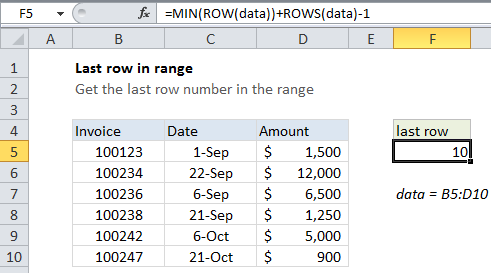## Excel Office

Excel How Tos, Tutorials, Tips & Tricks, Shortcuts

# Last row number in range

You can get the last row in a range with a formula based on the ROW function. See example below;

## Formula

`=MIN(ROW(range))+ROWS(range)-1`## Explanation

In the example shown, the formula in cell F5 is:

`=MIN(ROW(data))+ROWS(data)-1`

where data is a named range for B5:D10

### How this formula works

When given a single cell reference, the ROW function returns the row number for that reference. However, when given a range that contains multiple rows, the ROW function will return an array that contains all row numbers for the range.

Also See:   How to get first column number in range in Excel

If you want only the first row number, you can use the MIN function to extract just the first row number, which will be the lowest number in the array.

Once we have the first row, we can just add the total rows in the range and subtract 1, to get the last row number.

Also See:   Position of first partial match in Excel

### Simple version

When a formula returns an array result, Excel will display the first item in the array if the formula is entered in a single cell. This means that in practice, you can often just use a simplified version of the formula:

`=ROW(data)+ROWS(data)-1`

However, inside formulas, it can be necessary to make sure you are dealing with only one item, and not an array. In that case, you’ll want to use the the MIN or INDEX version below.

Also See:   Approximate match with multiple criteria in Excel

### Index version

Instead of MIN, you can also use INDEX to get the last row number:

`=ROW(INDEX(data,1,1))+ROWS(data)-1`

This is possibly a bit faster for large ranges, since INDEX just supplied a single cell to ROW.